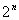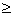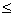Instruction for optimizing the parameters of a biochemical network by using a RS and a GA

1. Introduction

2003. 8. 1

# 1. Introduction

This search engine optimizes the parameters of biochemical networks by using a random search (RS) and a genetic algorithm (GA).

# 2. A flow of processes for program execution

The CADLIVE system converts a concrete biochemical network into a mathematical model, resulting in generating the user functions that are written in C language. In order to optimize the parameters for the biochemical network model, CADLIVE compiles the user functions to link to the library of random searches (RSs) and GAs, resulting in producing a load module on LINUX or UNIX. This section explains how the user functions are linked to the libraries of random searches and GAs to create the load module.

The main procedure is:

(1)　Define the user functions, which can be created by the CADLIVE system.

(2)　Describe "Makefile", and make a load module.

(3)　Edit the various files that are necessary for RSs and GAs.

# 3. Definition of user functions

## 3.1 Files for a parameter search

Users make their working directory under the directory of /home/LifeSimulator/TEST/Tools/examples. In order to make a load module, two include files and three user functions are required as follows.

1. life.h

2. lsUsrFitness.h

3. void usr_fvec(double fvec[], double y[], double Gene, Parameter *p)

4. void usr_fjac(double fjac[][MAX_VAR_NUM], double y[], double Gene, Parameter *p)

5. int LsUsrFitness(double **y, int num_row, int num_col, int ls_ret, double *fitness)

## 3.2 How to describe "LsUsrFitness function"

Users have to define three functions. The detailed description for two functions of "usr_fvec" and "usr_fjac", which are defined in "life.h", are explained in the instruction of the checkdae module. Here, we explain the "LsUsrFitness" function, where the indexes and the return value are defined as follows.

Return value:

0 (Normal), -1(Abnormal, the function is terminated abnormally)

Index:

double **y              Solution matrix: time evolution of concentrations (input)

int num_row          the number of rows (input)

int num_col           the number of columns(input)

int ls_ret                a return value from a simulator (input)

double *fitness       fitness(output)

Users are able to define the fitness using the time-course data. The 0-th column of the solution matrix y indicates time, and i-th column the time evolution of y[i], as follows,

Time,      y,       y

1.000342, 0.097256, 0.289668

1.999686, 0.087374, 0.322411

2.999703, 0.077780, 0.362159.

The index of "ls_ret" is the return code that the Simulator (CADLIVE) gives, depending on the result of the simulation under the given parameters by GAs or RSs. The return code of –1 indicates that the batch simulation terminates abnormally and its simulated results are abandoned. If users want to terminate the program when such a batch simulation fails, set the return value of "LsUsrFitness" as –1. In contrast, if users want to continue searching despite the return code (ls_ret) of –1 from a batch simulation, users put an appropriate fitness value (which is should be far distant from an optimal value) and enforce the "LsUsrFitness" function to return zero. In the case of the steady-state solver, time is put as zero.

## 3.3 Example for user function: heat shock response

We show how to define the user functions by referring an example. The fitness is defined as the total of the solution matrix when a batch simulation terminates normally (the return value is zero). On the other hand, the fitness defined as –100 (which should be far distant from optimal values), when a batch simulation terminates abnormally (the return code is –1). Consequently, with the increase in the generation, the inappropriate parameter sets disappear. The use of a random search can determines the region where the solutions may exist.

#include "life.h"

#include "lsUsrFitness.h"

int     LsUsrFitness(double **y, int num_row, int num_col, int ls_ret, double *fitness)

{

int     i, j;

if(ls_ret == -1){

*fitness = -100;

goto out;

}

*fitness = 0;

for(i = 0; i < num_row; i++){

for(j = 0; j < num_col; j++){

*fitness += y[i][j];

}

}

out:

return 0;

}

The other functions,

void usr_fvec(double fvec[], double y[], double Gene, Parameter *p),

void usr_fjac(double fjac[][MAX_VAR_NUM], double y[], double Gene, Parameter *p),

are defined by the CADLIVE Simulator (checkdae module). The details are explained elsewhere.

4. Define Makefile

In the same directory where the user functions are defined, edit "Makefile". The following box shows the example, where users edit "Makefile" for generating a load module for a RS (or a GS). Users set the name of the load module and that of user’s file as BIN and SRC, respectively. To make a load module for GAs, replace \$(RS_LIB) by \$(GA_LIB).

#

#       make for HeatShock(Random Search)

#

TOP     = ../..　　　                     　# A change is impossible

include \$(TOP)/makedef/make_def　　　　　  # A change is impossible

BIN     =  name of load module (e. g.,HeatShock)

SRC     =  name of user function (e.g.,heatshock_usrfunc.cpp)

MY_INC  = \$(INC)　　　　　　　　　　　　　　　   # A change is impossible

MY_LIB  = \$(RS_LIB) \$(SOLV_LIB) \$(UTIL_LIB) \$(MPI_LIB)

# For GA, \$(GA_LIB) replaces \$(RS_LIB)

include \$(TOP)/makedef/make_exec　　　   # A change is impossible

Following editing "Makefile", do make. The load module is named as the same one that users have defined as BIN.

# 5. Description of parameter files and program execution

The execution of a RS requires the parameter file that the checkdae module generates (The checkdae module is installed in the CADLIVE simulator). If the parameter file is not available, instead of it, the parameter-range setting file determines the ranges of the variables to search and the values of the fixed parameters.

The execution of a GA requires not only the parameter-range setting file (or parameter file), but also

GA-parameter setting file (that sets the control parameters regarding GAs), and

GA-population setting file (that sets the initial values of the population).

For an option, the parameter-range setting file that sets the ranges of the variables to search can be employed instead of the parameter file.

## 5.1 Description of parameter file

Users have to reedit the parameter file to optimize parameters by a RS and GA. we refer to instruction of how to reedit the parameter file.

In the parameter file for a RS and GA,

l         P_SURVEY must be zero.

l         The use of "parallel computation" is invalid. Use the mpirun command.

l         PARAM must be described as follows.

PARAM ; name ; index ; setting value ; [starting value] ; number of parameter survey ; D/R/S ; changing value ; lower value ; upper value ; tag for identifying parameters ;  # comment

Choose S out of D/R/S with respect to the parameter to search. For the parameters that are not searched, their values are fixed despite the value of D/R (The set of D/R is invalid). The constant players cannot be the parameters to search. Input the upper and lower values, which must be put for both GAs or RSs. The number of parameter survey and the changing value are invalid (for both GAs and RSs). The number of parameter survey must be set on a command line. The other respects are the same as described in the CADLIVE Simulator.

## 5.2 GA-parameter setting file

The execution of GAs requires not only the parameter file (or parameter-range setting file), but also the GA-parameter setting file and the GS-population setting file. The GA-parameter setting file is provided by:

# Format of GA-parameter setting file

Key word; Alternatives; parameter_1; ……. ; parameter_n;　　# comments

, where # indicates comments. The key words and alternatives are selected from the strings shown in Table 1.

Table 1 Control parameters for setting GAs. Key words and their alternatives are selected. Upper and lower cases are distinguished for defining the parameters. The program runs until the fitness becomes more than the value for terminating a search. Both RGA and BGA can select the transparent alternatives. The thin gray alternatives can be selected only by RGA, and the dark gray alternatives can be selected only by BGA.

 Key word Alternatives Meaning ENCODE REAL Real GA (Encode method) BINARY Binary coding bit string type GA GRAY Gray coding bit string type GA GATYPE DGA Distributed GA (island model) (Island model) DIGA Distributed and Integrated GA DIGENESIS NORMAL Normal generation (Generation alternation) MGG MGG CROSSOVER BLX Blend crossover (Crossover method) UNDX Unimodal Normal Distribution crossover UNDXm Multi-parental Unimodal Normal Distribution crossover SPX Symplex crossover NPOINTS n-point crossover (BGA) NONE No crossover MUTATION RegionUni Uniform mutation within region (Mutation method) FixedUni Uniform mutation with fixed width FixedNormal Normal mutation with fixed width VariableUni Uniform mutation with variable width VariableNormal Normal mutation with variable width BitReverse Bit reverse mutation NONE None

Users can set the parameters with respect to each alternative in the following box.

# Parameter description with respect to key words and alternatives.

ENCODE; REAL;

ENCODE; BINARY; quantization number;

ENCODE; GRAY; quantization number;

GATYPE; DGA; maximum generation number; value for terminating a search; number of islands; population number within islands; immigration method; immigration interval; immigration rate;

(The final two parameters are required for immigration operation. Actually the above parameters must be on one line.)

GATYPE; DIGA; maximum generation number; value for terminating a search;  number of islands; population number within islands; generation number for integration;

DIGENESIS; NORMAL; number of elites; rule for selection; tournament size (if tournament is employed);

DIGENESIS; MGG; number of children generated;

CROSSOVER; BLX; alpha;

CROSSOVER; UNDX; alpha; beta;

CROSSOVER; UNDXm; alpha; beta; m;

CROSSOVER; SPX; epsilon;

CROSSOCER; NPOINTS; n;

CROSSOVER; NONE;

MUTATION; FixedUni; mutation rate; width of parameter range;

MUTATION; FixedNormal; mutation rate; standard deviation;

MUTATION; VariableUni; mutation rate;

MUTATION; VariableNormal; mutation rate;

MUTATION; RegionUni; mutation rate;

MUTATION; BitReverse; mutation rate;

MUTATION; NONE;

In the bit-string GA, the real values are converted into the bit sequences by dividing the range of the variables with. The number of n that determines the resolution is named as the quantization number. A part of the parameters for GA type, generation alternation, crossover, and mutation depends on the parameters of the encode methods. The parameters for GA type, generation alternation, crossover, and mutation are independent mutually.

Table 2 Values where users are allowed to set with respect to each parameter. Note that the GA module can distinguish the upper or lower cases of strings.

 Parameter Values Default value quantization number Integer1 1 maximum generation number Integer1 100 value for terminating a search real value (double type) -1.00E-10 number of islands Integer1 3 population number within islands variable number +2IntegerMaximum 30 immigration switch ON, OFF ON immigration interval Integer1 3 immigration rate Real value [0,1] 1 generation for integration Integer1 5 number of elites Integer0 population number selection rule Roulette, Tournament, Random Tournament size of tournament 1Integer(population number of islands – number of parents +1) 2 number of children generated by MGG 1IntegerMaximum population number of islands alpha (BLX) Real value > 0 0.5 alpha (UNDX) Real value > 0 1 alpha (UNDXm) Real value > 0 1 beta (UNDX) Real value > 0 0.35 beta (UNDXm) Real value > 0 0.35 M 1Integernumber of variables max(1, the number of variables -2) epsilon Real value > 0 1/sqrt (number of variables  +2) N Integer1 1 mutation rate Real value [0,1] 1/ number of variables width Real value > 0 20 standard deviation Real value > 0 2

## 5.3 GA-population setting file

The random search produces the result file that the GA module is able to read as a GA-population setting file from. In the n-dimensional parameter space, the GA-population setting file is provided by:

# Format for GA-population setting file

SearchID, fitness, parameter1, ....., , parameter_n

....................................

**

SearchID, fitness, parameter1, ....., , parameter_n

The symbol of "#" indicates that the line is comments.

The section between islands is expressed by " **". Users neither have to put " **" on the initial island nor under the final island. If such descriptions are employed, an empty island is regarded to exist.

## 5.4 Execution of program

Random search

Execution on a command line

%load module name　–I[parameter file]　 –O[RS result file] –n total number of random searches　–j  number of jobs that the master CPU requires  –r

Bracketed sections are files (don't type the [] characters).

For help,

Options

-I[file name]: (required) parameter file

-O[file name] :(required) RS result file. The details are explained later.

-n[integer]:(required)  total number of random searches.

-j[integer]:(option, valid for MPI )  number of jobs that the master CPU requires. For a single, -j option is invalid. The default value for MPI is one.

-r :(option) With this option, the list of parameters in the RS result file is not sorted. Without it, the list of result file is sorted to output. The parameters are sorted separately every island, but the total parameters are not sorted.

RS result files

For a single process, two RS result files are output.

(file name)

(file name)_list

The parenthesed part is the name of file ( () is not output).

For MPI, the number of the RS result files is (number of slaves + one).

(file name)_(rank of process)  Except the rank of 0.

(file name)_list

The parenthesed part is the name of file ( () is not output).

The former file outputs the result of simulation, the fitness, and the values of the parameters to search. The latter one writes the fitness and the values of the parameters.

Search by GAs

Execution on a command line

%Load module name　–I[parameter file]　–p[GA-parameter setting file]　–s[GA-population setting file]　–f[GA result file]

Bracketed sections are files (don't type the [] characters).

Options

-I[file name]: (required) parameter file, which the checkdae program generates and can be read by the CADLIVE simulator and by the GA modules.

-p[file name]: (required) GA-parameter setting file.

-s[file name]: (option) GA-population setting file.

-f[file name]: (option) GA result file that output the fitness and the values of the searched parameters of the entire individuals in the whole islands every generation

-h : Show help.

Execution by MPI for (RSs and GAs)

When MPI is used, put “mpirun –np [number of processes]” on the top of the load module name.

% mpirun –np <number of process> <load module name>　<arguments>

%mpirun –np 3 <load module name> –r domain.dat -p param.dat  -f output.dat

When the MPICH-G is used, put “mpirun –glubusrsl [rsl file name] on the top. To learn how to edit the "**.rsl" file, refer to the manuals for MPICH-G by:

%mpirun –globusrsl <rsl file>

The "rsl" file is described in the manual of MPICH-G.

One process for one CPU is recommended.